Precalculus : Sums of Infinite Series

Example Questions

Example Question #1 : Finding Sums Of Infinite Series

Find the value for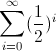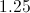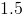Explanation:

To best understand, let's write out the series. So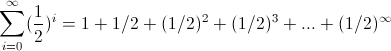We can see this is an infinite geometric series with each successive term being multiplied by.

A definition you may wish to remember is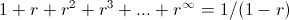where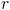stands for the common ratio between the numbers, which in this case isor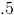. So we get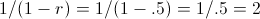Example Question #1 : Sums Of Infinite Series

Evaluate: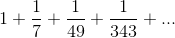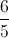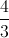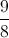The series does not converge.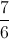Explanation:

This is a geometric series whose first term is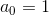and whose common ratio is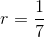. The sum of this series is: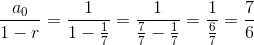Example Question #1 : Sums Of Infinite Series

Evaluate: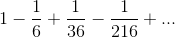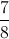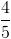The series does not converge.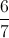Explanation:

This is a geometric series whose first term isand whose common ratio is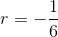. The sum of this series is: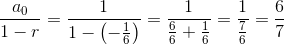Example Question #34 : Sequences And Series

What is the sum of the following infinite series?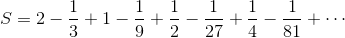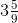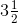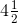divergesExplanation:

This series is not alternating - it is the mixture of two geometric series.

The first series has the positive terms.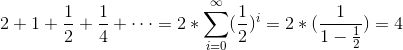The second series has the negative terms.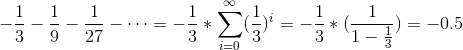The sum of these values is 3.5.

Example Question #35 : Sequences And Series

What is the sum of the alternating series below?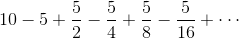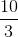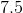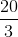Explanation:

The alternating series follows a geometric pattern.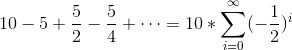We can evaluate the geometric series from the formula.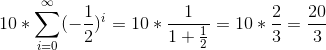Example Question #1 : Sums Of Infinite Series

Find the sum of the following infinite series: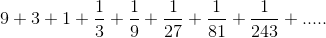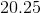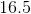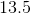Explanation:

Notice that this is an infinite geometric series, with ratio of terms = 1/3. Hence it can be rewritten as: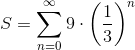Since the ratio, 1/3, has absolute value less than 1, we can find the sum using this formula: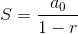Where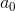is the first term of the sequence. In this case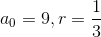, and thus: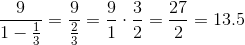All Precalculus Resources